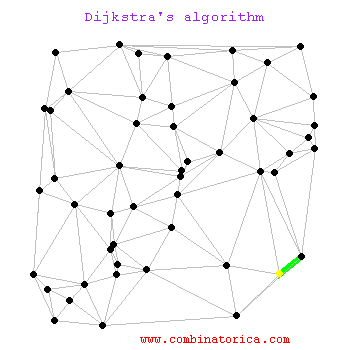## Sunday, April 29, 2018

### Djikstra's and Bellman-Ford's Shortest Path Algorithms

Let's discuss some path-finding algorithms!

In this blog post we are going to look at two types of algorithms for traversing graphs: the Dijkstra and Bellman-Ford algorithms. Let's begin by defining single-source shortest path algorithms, describing each, then we will move into a comparison, and finally we will end with some pseudo-code to aid with your implementation.Singe-source shortest path algorithms (SSSP) work within graphs and seek to find the shortest path between any two given vertices. The shortest path can be decided by the number of edges travelled (the distance) or by the sum of the weighted nodes. As the algorithm continues, it finds shorter and shorter paths and updates the adjacent node's path to the shortest one possible; that is to say, the algorithm defines each vertex's distance from the start vertex as well as its preceding pointer. An interesting idea behind SSSP algorithms is that a programmer can find the shortest path from one node to all the nodes in a graph as easily as the distance to just one of them. This is an unexpected but useful result.

Dijkstra's and Bellman-Ford's Algorithms thus must sound quite familiar to one another, and in fact they are. Dijkstra's algorithm starts from a source node and each iteration appends a vertex to the SSSP tree that has been created. Dijkstra's algorithm sets up two sets of vertices in the graph: those that have had their paths determined and those that have yet to be reached. As the algorithm continues, distances are compared and thus all the vertices are moved from the latter list onto the finalized SSSP tree. This visualization shows the formation of that shortest path tree.As aforementioned, this is a very similar mechanism to the Bellman-Ford algorithm. A key difference is that the Bellman-Ford Algorithm is capable of handling negative weights whereas Dijkstra's algorithm can only handle positive weights. This makes the Bellman-Ford algorithm applicable for a wider range of input graphs.

Let's go over some pseudocode for both algorithms.

SSSP Algorithm Steps

1. Initialize the cost of each node to infinity
2. Initialize the cost/distance of the source to 0
3. Where there are un-visited vertices left in the graph
1. Select an unknown node b with the lowest cost, and set that b to known.
4. For each node a that is adjacent to b,
1. a's cost = minimum of (a's old cost, b's cost + cost (b, a))
Dijkstra's Algorithm Pseudocode

Dijkstra(startVertex) {
for each vertex in the graph {
currentVertex-> distance = infinity
currentVertex-> preceding = 0
push the currentVertex to the unvisited list
}

startVertex's distance from itself = 0

while (unvisitedList is not empty) {
currentVertex = popmin from unvisitedList

for each Vertex adjacent to currentVertex {
edgeWeight = weight of edges from the currentVertex
alternate = currentVertex-> distance + edgeWeight

if (alternate < adjacentVertex's distance) {
}
}
}
}

Bellman-Ford's Algorithm Pseudocode
BellmanFord(startVertex) {
for each vertex in the graph {
currentVertex-> distance = infinity
currentVertex-> preceding = 0
}

startVertex's distance from itself = 0

for i = 1 until (numberOfVertices - 1) {
for each adjacentVertex for currentVertex {
edgeWeight = sum edges from adjacent to currentVertex
alternate = currentVertex-> distance + edgeWeight

if alternate < adjacentVertex's distance {